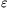Mathematical and Physical Journal
for High Schools
Issued by the MATFUND Foundation
 Already signed up? New to KöMaL?

#Problem A. 578. (January 2013)

A. 578. For every integer n2 let P(n) be the product of all expressions of the formwhere the signs of the terms are chosen arbitrarily.

(a) Prove that P(n) is a positive integer.

(b) Prove that for all>0 there exists an n0 such that for every n>n0 the largest prime divisor of P(n) is smaller than.

(5 pont)

Deadline expired on February 11, 2013.

### Statistics:

 10 students sent a solution. 3 points: 2 students. 1 point: 8 students.

Problems in Mathematics of KöMaL, January 2013